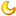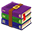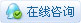##用户名 Email 自动登录 找回密码 密码 注册
 搜索

# 51单片机鱼缸温度控制器（程序以及protues仿真在压缩包里）

425主题 296积分296发表于 2020-1-21 17:43:04 | 显示全部楼层 |阅读模式
 随着人们生活水平的不断提高，目前各式各样的观赏鱼缸之类的工艺产品逐渐进入了家庭和宾馆、商场等公共场所,由于现有的观赏鱼缸的水温检测、水温控制等操作都特别繁琐，给人们带来了很大的不便。本文通过对目前大多数鱼缸控制设备应用现状的分析和研究,提出了一种鱼缸温度智能检测系统的设计方案。针对目前大多数鱼缸控制设备价格昂贵、安装繁琐、运行费用高,一般的用户难以使用的情况,我们结合单片机强大的开发技术,设计制作了一种以单片机为控制核心传感器技术进行温度显示并实现智能温度检测。此系统主要以STC89C51单片机检测系统为核心实现对鱼缸的集中控制和管理，能够对鱼缸温度进行自动检测，温度实时显示用LCD显示屏实现，用DS18B20温度传感器完成实时传送温度的指标。 仿真原理图如下（proteus仿真工程文件可到本帖附件中下载）智能鱼缸.zip (113.09 KB, 售价: 1 工控币) 2020-1-21 17:35 上传 点击文件名下载附件#include #define uint unsigned int #define uchar unsigned char           //宏定义 #define LCD1602 P0 sbit SET=P3^1;                            //定义调整键 sbit DEC=P3^2;                            //定义减少键 sbit ADD=P3^3;                            //定义增加键 sbit BUZZ=P3^6;                            //定义蜂鸣器 sbit ALAM=P1^2;                                //定义灯光报警 sbit ALAM1=P1^4; sbit DQ=P3^7;                             //定义DS18B20总线I/O         sbit RS = P2^7; sbit EN = P2^6; bit shanshuo_st;                            //闪烁间隔标志 bit beep_st;                                     //蜂鸣器间隔标志 uchar x=0;                                      //计数器 uchar code tab1[]={"Now Tem:   .  C "}; uchar code tab2[]={"TH:   C  TL:   C"}; uint c; uchar Mode=0;                             //状态标志 signed char TH=40;                  //上限报警温度，默认值为40 signed char TL=10;                   //下限报警温度，默认值为10 //============================================================================================ //====================================DS18B20================================================= //============================================================================================ /*****延时子程序*****/ void Delay_DS18B20(int num) {   while(num--) ; } void delay(uint xms)//延时函数，有参函数 {         uint x,y;         for(x=xms;x>0;x--)          for(y=110;y>0;y--); } /*****初始化DS18B20*****/ void Init_DS18B20(void) {   unsigned char x=0;   DQ = 1;         //DQ复位   Delay_DS18B20(8);    //稍做延时   DQ = 0;         //单片机将DQ拉低   Delay_DS18B20(80);   //精确延时，大于480us   DQ = 1;         //拉高总线   Delay_DS18B20(14);   x = DQ;           //稍做延时后，如果x=0则初始化成功，x=1则初始化失败   Delay_DS18B20(20); } /*****读一个字节*****/ unsigned char ReadOneChar(void) {   unsigned char i=0;   unsigned char dat = 0;   for (i=8;i>0;i--)   {     DQ = 0;     // 给脉冲信号     dat>>=1;     DQ = 1;     // 给脉冲信号     if(DQ)     dat|=0x80;     Delay_DS18B20(4);   }   return(dat); } /*****写一个字节*****/ void WriteOneChar(unsigned char dat) {   unsigned char i=0;   for (i=8; i>0; i--)   {     DQ = 0;     DQ = dat&0x01;     Delay_DS18B20(5);     DQ = 1;     dat>>=1;   } } /*****读取温度*****/ unsigned int ReadTemperature(void) {   unsigned char a=0;   unsigned char b=0;   unsigned int t=0;   float tt=0;   Init_DS18B20();   WriteOneChar(0xCC);  //跳过读序号列号的操作   WriteOneChar(0x44);  //启动温度转换   Init_DS18B20();   WriteOneChar(0xCC);  //跳过读序号列号的操作   WriteOneChar(0xBE);  //读取温度寄存器   a=ReadOneChar();     //读低8位   b=ReadOneChar();    //读高8位   t=b;   t<<=8;   t=t|a;   tt=t*0.0625; // t= tt*10+0.5;     //放大10倍输出并四舍五入   t= tt*10+0.5;   return(t); } /*****读取温度*****/ void check_wendu(void) {         c=ReadTemperature()-5;                          //获取温度值并减去DS18B20的温漂误差         if(c>1200)         c=1200; } /********液晶写入指令函数与写入数据函数，以后可调用**************/ void write_1602com(uchar com)//****液晶写入指令函数**** {         RS=0;//数据/指令选择置为指令 //        rw=0; //读写选择置为写         LCD1602=com;//送入数据         delay(1);         EN=1;//拉高使能端，为制造有效的下降沿做准备         delay(1);         EN=0;//en由高变低，产生下降沿，液晶执行命令 } void write_1602dat(uchar dat)//***液晶写入数据函数**** {         RS=1;//数据/指令选择置为数据 //        rw=0; //读写选择置为写         LCD1602=dat;//送入数据         delay(1);         EN=1; //en置高电平，为制造下降沿做准备         delay(1);         EN=0; //en由高变低，产生下降沿，液晶执行命令 } void lcd_init()//***液晶初始化函数**** {         uchar a;         write_1602com(0x38);//设置液晶工作模式，意思：16*2行显示，5*7点阵，8位数据         write_1602com(0x0c);//开显示不显示光标         write_1602com(0x06);//整屏不移动，光标自动右移         write_1602com(0x01);//清显示         write_1602com(0x80);//日历显示固定符号从第一行第1个位置之后开始显示         for(a=0;a<16;a++)         {                 write_1602dat(tab1[a]);//向液晶屏写日历显示的固定符号部分                 delay(3);         }         write_1602com(0x80+0x40);//时间显示固定符号写入位置，从第2个位置后开始显示         for(a=0;a<16;a++)         {                 write_1602dat(tab2[a]);//写显示时间固定符号，两个冒号                 delay(3);         } } void display() {         if(Mode==0)         {                 write_1602com(0x80+8);                 write_1602dat(c/1000+0x30);                 write_1602dat((c%1000)/100+0x30);                 write_1602dat(((c%1000)%100)/10+0x30);                 write_1602com(0x80+12);                 write_1602dat(((c%1000)%100)%10+0x30);                 write_1602com(0x80+13);                 write_1602dat(0xdf);                 write_1602com(0x80+0x40+3);                 write_1602dat(TH/10+0x30);                 write_1602dat(TH%10+0x30);                 write_1602dat(0xdf);                 write_1602com(0x80+0x40+12);                 write_1602dat(TL/10+0x30);                 write_1602dat(TL%10+0x30);                 write_1602dat(0xdf);                                 }                                                                   } //===================================================================================== /*****初始化定时器0*****/ void InitTimer(void) {         TMOD=0x1;         TH0=0x3c;         TL0=0xb0;     //50ms（晶振12M）         EA=1;      //全局中断开关         TR0=1;         ET0=1;      //开启定时器0 } void KEY() {                         //功能键         if(SET==0)         {                 BUZZ=0;                 delay(10);                 if(SET==0)                 {                         Mode++;                         if(Mode==3)                         Mode=0;                         BUZZ=1;                 }                 while(SET==0)                 {                         if(Mode==0)                                 {                                 //        write_1602com(0x80+0x40+6);                                         write_1602com(0x0c);                                 }                                 else if(Mode==1)                                 {                                         write_1602com(0x80+0x40+4);                                         write_1602com(0x0f);                                 }                                 else                                 {                                         write_1602com(0x80+0x40+13);                                         write_1602com(0x0f);                                 }                                                                         }         }         //增加         if(ADD==0&&Mode==1)         {                 BUZZ=0;                 delay(10);                 if(ADD==0)                         {                         TH++;                         if(TH>=99)                                 TH=99;                         write_1602com(0x80+0x40+3);                         write_1602dat(TH/10+0x30);                         write_1602dat(TH%10+0x30);                         write_1602com(0x80+0x40+4);                                 BUZZ=1;                 }                 while(ADD==0);                         }         //减少         if(DEC==0&&Mode==1)         {                 BUZZ=0;                 delay(10);                 if(DEC==0)                 {                         TH--;                         if(TH==TL)                                 TH=TL+1;                         write_1602com(0x80+0x40+3);                         write_1602dat(TH/10+0x30);                         write_1602dat(TH%10+0x30);                         write_1602com(0x80+0x40+4);                                 BUZZ=1;                 }                 while(DEC==0);         }         if(ADD==0&&Mode==2)         {                 BUZZ=0;                 delay(10);                 if(ADD==0)                         {                         TL++;                         if(TL==TH)                                 TL=TH-1;                         write_1602com(0x80+0x40+12);                         write_1602dat(TL/10+0x30);                         write_1602dat(TL%10+0x30);                         write_1602com(0x80+0x40+13);                                 BUZZ=1;                 }                 while(ADD==0);                         }         //减少         if(DEC==0&&Mode==2)         {                 BUZZ=0;                 delay(10);                 if(DEC==0)                 {                         TL--;                         if(TL<=0)                                 TL=0;                         write_1602com(0x80+0x40+12);                         write_1602dat(TL/10+0x30);                         write_1602dat(TL%10+0x30);                         write_1602com(0x80+0x40+13);                                 BUZZ=1;                 }                 while(DEC==0);                        } } /*****报警子程序*****/ void Alarm() {         if(x>=10){beep_st=~beep_st;x=0;}         if(Mode==0)         {                 if((c/10)>=TH)                 {                         ALAM=0;                         ALAM1=1;                         if(beep_st==1)                         BUZZ=0;                         else                         BUZZ=1;                 }                 else if((c/10)

 本版积分规则 回帖后跳转到最后一页|小黑屋|手机版|工控技术网 |网站地图

GMT+8, 2020-10-29 12:22